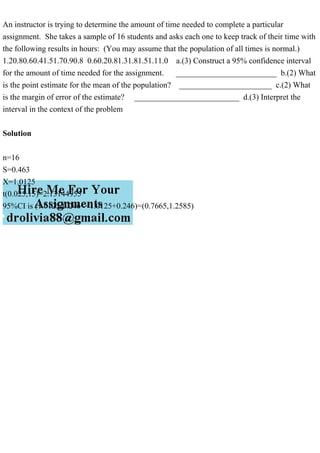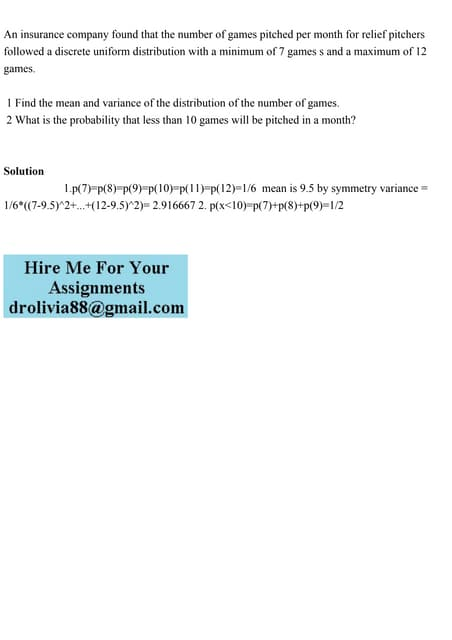Publicité

# An instructor is trying to determine the amount of time needed to co.pdf

25 Mar 2023Prochain SlideShareAn insurance company found that the number of games pitched per mont.pdf
Chargement dans ... 3
1 sur 1
Publicité

### An instructor is trying to determine the amount of time needed to co.pdf

1. An instructor is trying to determine the amount of time needed to complete a particular assignment. She takes a sample of 16 students and asks each one to keep track of their time with the following results in hours: (You may assume that the population of all times is normal.) 1.20.80.60.41.51.70.90.8 0.60.20.81.31.81.51.11.0 a.(3) Construct a 95% confidence interval for the amount of time needed for the assignment. _________________________ b.(2) What is the point estimate for the mean of the population? _______________________ c.(2) What is the margin of error of the estimate? __________________________ d.(3) Interpret the interval in the context of the problem Solution n=16 S=0.463 X=1.0125 t(0.025,15)=2.13144955 95%CI is (1.0125-0.246<<1.0125+0.246)=(0.7665,1.2585)
Publicité